# 修改Xshell代码配色和背景水印 提高代码个性化和版权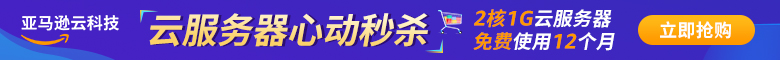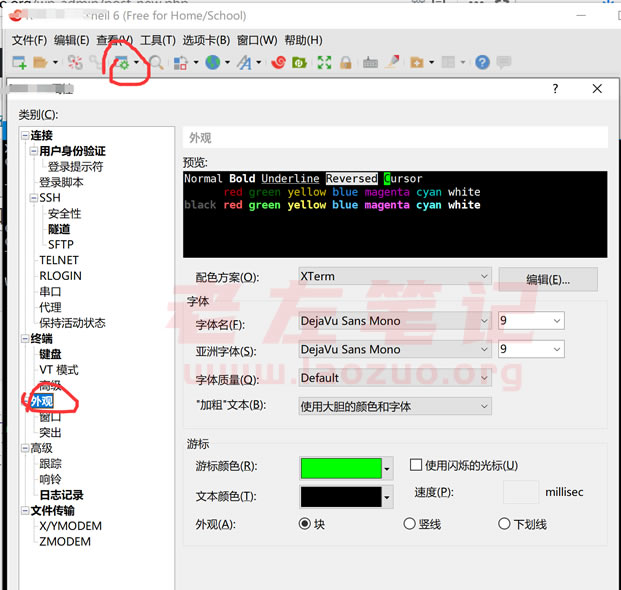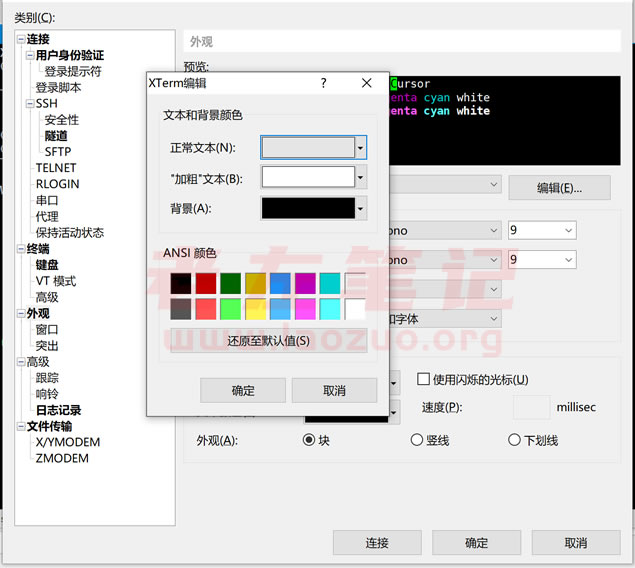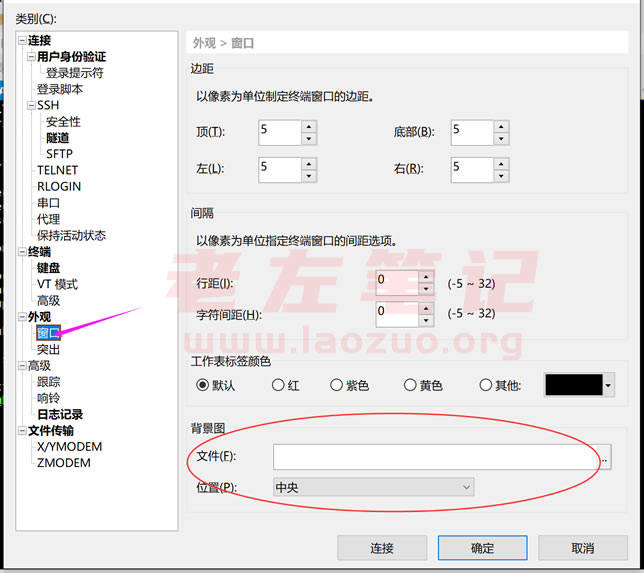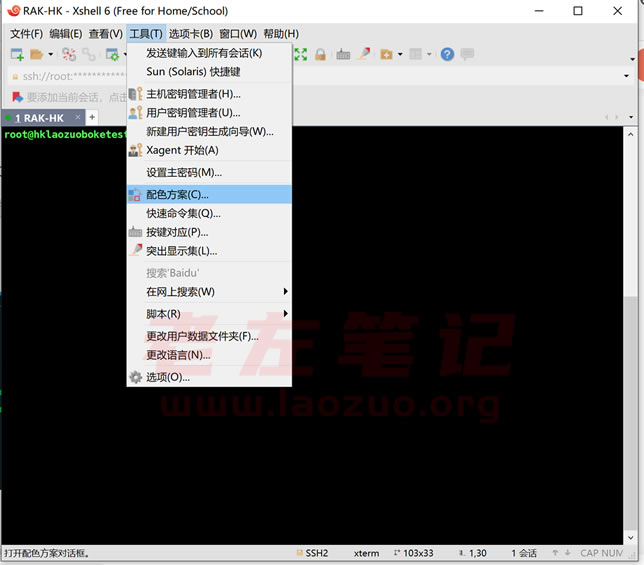1、配色1

[ubuntu] text(bold)=ffffff
text=ffffff
white(bold)=eeeeec
green=4e9a06
red(bold)=ef2929
green(bold)=8ae234
black(bold)=555753
red=cc0000
blue=3465a4
black=000000
blue(bold)=729fcf
yellow(bold)=fce94f
cyan(bold)=34e2e2
yellow=c4a000
magenta=75507b
background=300a24
white=d3d7cf
cyan=06989a
[Names] count=1
name0=ubuntu

2、配色2

[Solarized Dark] text=00ff40
cyan(bold)=93a1a1
text(bold)=839496
magenta=dd3682
green=859900
green(bold)=586e75
background=042028
cyan=2aa198
red(bold)=cb4b16
yellow=b58900
magenta(bold)=6c71c4
yellow(bold)=657b83
red=dc322f
white=eee8d5
blue(bold)=839496
white(bold)=fdf6e3
black=002b36
blue=268bd2
black(bold)=073642
[Names] name0=Solarized Dark
count=1